# Introduction

Video Transcript

There are four modules in the Introduction to the Revised Mathematics TEKS professional development series. Each module is approximately three hours. Each module gives you the opportunity to focus on the TEKS for one of the following high school mathematics courses: Algebra I, Geometry, or Algebra II.

This is the second of four modules to introduce the revised mathematics TEKS. The four modules are

• The Revised Math TEKS (Grades 9–12) with Supporting Documents,
• Applying the Mathematical Process Standards,
• Completing the Gap Analysis, and
• Achieving Fluency and Proficiency.

# Exploring the Mathematical Process Standards

Watch the video below for an overview of the mathematical process standards.

Watch the video below to frame considerations regarding the mathematical process standards. Explore the process standards in the Vertical Alignment Chart that includes Algebra I and Algebra II. Examine the mathematical process standards through the lens of four big ideas:

• Communication
• Reasoning, generalizing, and problem solving
• Analysis
• Modeling, using tools and connecting to representations

Find evidence in the mathematical process standards that supports these big ideas, and record your findings on the Exploring the Mathematical Process Standards journal page.

Is there a distinct separation between these four big ideas? Why or why not?

Watch the video below for possible reponses to this question.

# Mathematical Process Standards Summary

Kindergarten through grade 8 applied process skills through the Underlying Processes and Mathematical Tools strand prior to our TEKS revision and applied the mathematical process standards that you have just studied when they implemented their revised TEKS.

Write a three sentence summary of the mathematical process standards on the Exploring The Mathematical Process Standards (continued) page in your journal.

How might the mathematical process standards support learning in your classroom?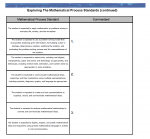# Building Depth and Complexity

Video Transcript

How does this graphic capture the intent behind the revised mathematical process standards?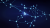Watch the video below and consider this question:

What role do the mathematical process standards play when "amplifying" an instructional task?

Watch the video below and consider this question:

How are the revised mathematical process standards embedded in the considerations for amplifying a task from The Revised Math TEKS (Grades 9–12) with Supporting Documents module?

Watch the video below and consider the following question:

How are the revised mathematical process standards related in these considerations?

Watch the video below and consider these questions:

How do you take the needs of your students into consideration? When providing a task, how could you provide entry to the task that takes into account the needs of a struggling student? An English language learner? An advanced student? A struggling advanced English language learner?

Video Transcript

Complete the trivia activity to refresh your knowledge of the documents reviewed in The Revised Math TEKS (Grades 9-12) with Supporting Documents module.

# Cross-Disciplinary Instruction and the ELPS

Watch the video below.

Complete the Vocabulary Notes activity below or in your journal to define vocabulary related to meeting the needs of all students.

Watch the video below and consider the following questions:

• What is your comfort level with the ELPS?
• What is your comfort level with designing instruction that is cross-disciplinary?
• What is your comfort level with designing instruction that allows for multiple entry points?

Watch the final video below.

# Bringing It All Together

Watch the video below and consider the following question:

What are the implications for students' acquisition and demonstration of their mathematical understandings related to content and process standards?

Record your thoughts on the Mathematical Process Standards—My Understanding journal page.

After recording your thoughts, watch the video below for possible considerations.

# Digging Deeper into Amplified Tasks

Choose two separate tasks to study. Complete the matrix in your Examining Amplified Instructional Task journal pages.

# Exploring the Texas Gateway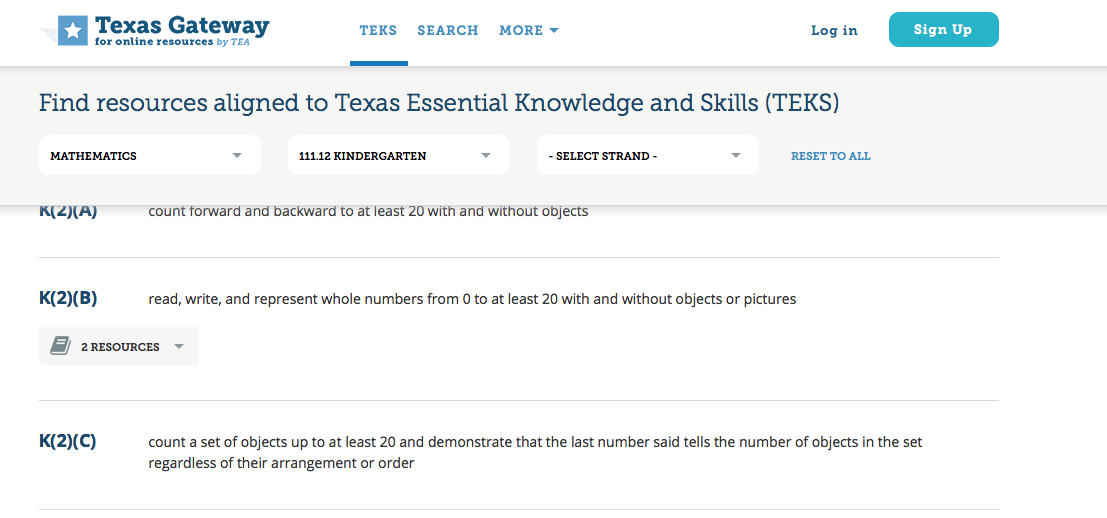Explore new resources found on the Texas Gateway and look for evidence of the mathematical process standards within the activities found on the Gateway. You can browse, use the faceted search, or search directly by TEKS.

The TEKS search is illustrated above. Select "Mathematics" and a course.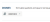This is an example from Algebra II. In the list of standards that result from your search, you will notice that some standards have a book icon and the the number of resources associated with that standard. Click to expand and see more information.

Click the title link of the resource to go to that resource.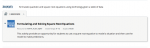Algebra I

• Linear Inequalities: A(3)(H)
• Linear Transformations: A(3)(E)

Geometry

• Introduction to Logical Reasoning: G(4)(B)
• Introduction to Probability: G(13)(C), G(13)(D)

Algebra II

• Absolute Value Inequalities: 2A(6)(A)
• Formulating and Solving Square Root Equations: 2A(4)(E), 2A(4)(F)

Complete the table in your Exploring the Texas Gateway journal page. Use the reflection questions provided below to guide your thinking. Record your thoughts in the My Reflections area of the journal page.

• How are the Mathematical Process Standards reflected in the Gateway activities? Give an example.
• With a particular Gateway activity in mind, how would you amplify the activity? Why?
• What was your favorite Gateway activity? Why?
• How could you use the Gateway activities with your students?
• What would you like to see more of? Why?
• What is your biggest take away from exploring the Gateway activities?